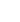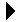# Simple List Recursion FunctionsCS 231 Lecture ExamplesDownload```; ; Common Lisp list functions. Many of these do the same thing as some ; built-in function. ; ; ; Return the length of a list. ; (defun len (lst) (if (null lst) 0 (+ 1 (len (cdr lst))) ) ) ; ; Total of the items of a list of numbers. ; (defun lsum (lst) (if (null lst) 0 (+ (car lst) (lsum (cdr lst))) ) ) ; ; Return the append of lst1 and lst2. ; (defun app (lst1 lst2) (if (null lst1) lst2 (cons (car lst1) (app (cdr lst1) lst2) ) ) ) ; ; Return the number of elements in list or any sublist. For a flat list, ; this is the same as the length: (members '(a b c)) is 3. For a list ; containing sublists, it counts the sublists, so ; (members '(a b (c d (e f)) g (h i))) is 9, while ; (len '(a b (c d (e f)) g (h i))) is 5. ; (defun members (lst) (cond ((null lst) 0) ((listp (car lst)) (+ (members (car lst)) (members (cdr lst)))) (t (+ 1 (members (cdr lst)))) ) ) ; ; Return the flat form of the list. That is, remove all nesting, and return ; the list of the same elements in the same order. So ; (A (B) (C (D E (F) G)) H) becomes (A B C D E F G H). ; (defun flatten (lst) (cond ((null lst) ()) ((listp (car lst)) (flatten (app (car lst) (cdr lst)))) (t (cons (car lst) (flatten (cdr lst)))) ) ) ; ; Remove each member of the list equal to the target. (defun pick_off (victim lst) (cond ((null lst) ()) ((equal victim (car lst)) (pick_off victim (cdr lst))) (t (cons (car lst) (pick_off victim (cdr lst)))) ) ) ```